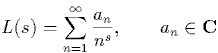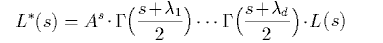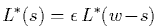ComputeL - Computing special values of L-functions
Tim Dokchitser

ComputeL is a pari package to compute special values of L-functions and their derivatives numerically to high accuracy. It is specifically designed to work with L-functions of motivic origin with an arbitrary number of Gamma-factors, not necessarily distinct. Current version 1.3.8 is implemented as a pari script. As an illustration, it includes examples of computations with

• Riemann zeta-function
• Dirichlet L-functions of Dirichlet characters and Dedekind zeta-function of a number field
• L-functions associated to (H1 of) curves of genus 1, 2, 3 and 4 over Q, in particular, Birch-Swinnerton-Dyer conjecture for the Buhler-Gross-Zagier elliptic curve of Mordell-Weil rank 3.
• L-functions associated to modular forms and Shintani's zeta-function

Short description

The computations are based on the algorithm from "Computing special values of motivic L-functions". The package applies to L-functions L(s) given by the usual series (for Re s>>0),Assume that L(s) multiplied by a suitable product of Gamma-factors,admits a meromorphic continuation to the whole of C. It has, moreover, at most simple poles and is known or conjectured to satisfy a functional equationor, slightly more generally, a functional equation relating L*(s) and a "dual" L-function.

To use the package one has to know the parameters of the functional equation (exponential factor A, sign e, weight w, Gamma-factor parameters l1...ld) and enough coefficients an. Note that there is no restriction on the number d of Gamma-factors and they do not have to be distinct. The poles of L*(s) have to be known as well, but not necessarily the residues in there. The functions provided allow to perform the following numerical computations with required precision:

• Determine the residues at the poles of  L*(s) if necessary
• Verify the assumed functional equation numerically
• For a given complex s, compute L(s) or its k-th derivative for a given k

Download the current version computel.zip (v1.3.8 July 2018). It's a zip archive and can be opened with "unzip -a computel.zip" under most operating systems. Alternatively download separate files below (all are pari scripts).

 computel - the package itself Basic examples ex-zeta - example: Riemann zeta function ex-chqua - example: L-function of a quadratic character (Legendre symbol) modulo odd prime p ex-nf - example: Dedekind zeta function of a number field ex-bsw - example: L-function of an elliptic curve and Birch-Swinnerton-Dyer conjecture ex-gen2 - example: L-function of a genus 2 curve ex-shin - example: Shintani's zeta function ex-eisen - example: Eisenstein series of weight k Additional examples ex-chgen - example: general Dirichlet character (functional equation involves two different L-functions) ex-delta - example: L-function of the modular form Delta of weight 12 (unusual coefficient growth) ex-zeta2 - example: Riemann zeta function for Im(s) large (precision issues) ex-gen3 ex-gen4 - example: L-functions of a genus 3 and a genus 4 curve    (precision issues when not enough coefficients are given)